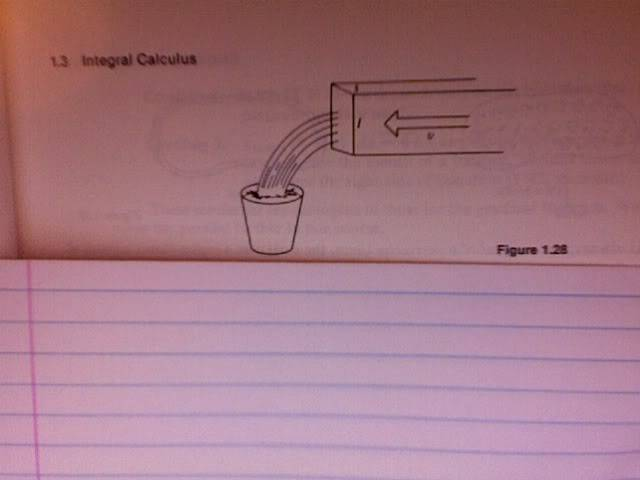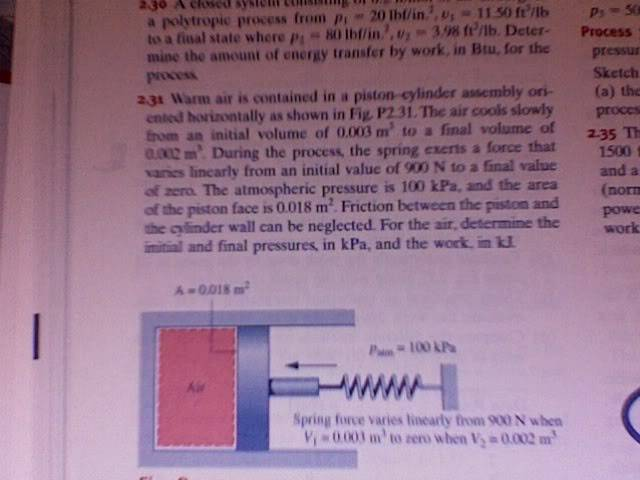# Average velocity vector question. Try again

## Homework Statement

A train with constant velocity of 60km/hour travels east for 40minutes; then in a direction 50degrees east of north for 20minutes; then west for 50minutes. A)What are the magnitude and B) angle of its average velocity during the trip?

## Homework Equations

sinx; cosx; tanx<-- I think.

## The Attempt at a Solution

My main trouble is finding out what the question is asking. I have determined that the magnitudes of each are 40km;20km;50km respectively.
I have drawn each vector on a cartesian coordinate plane such that they start at the origin and then connect tip to tail. IS the displacement vector the one that extends from the origin to the tip of the last one drawn?

This is my first post so I apologize if it is difficult to follow. Is there a way I could have drawn this w/out a scanner? Thanks.

Dick
Homework Helper
Your description is clear enough. And yes, the total displacement is just the vector connecting the starting point with the ending point.

Here is the work I have done, but the book is giving a solution of 7.9km/h....and 22.5 degrees east of north. I dont know where I went wrong. Am I approaching this all wrong?#### Attachments

Last edited:
berkeman
Mentor
The magnitude of the average vector velocity is the magnitude of the final displacement vector divided by the total time (and convert units to m/s as needed). The angle is the angle of your resultant V up from the horizontal (East) axis. I get about the right answer using the numbers from your picture -- did you account for the time correctly?

sorry...the solution was not in m/s...it is 7.9km/h.....The displacement vector is the dotted life correct? which equals 19.9. Divided by by 1 and 5/6 of an hour is getting me 10.8.
And the angle is stated as East OF North, meaning the angle formed by the Y axis and the vector(clockwise).

berkeman
Mentor
I think I see your error. See how it's initially 40+15.3km to the right, and then later 50km to the left? I had wondered where you were getting the 19 number from.....

19 is the length of hypoteneuse (the displacement vector) formed by the right triangle with a=15.3 and b=12.8.

Wow. You are correct in that regard. 55.3 east NOT 65.3.....Holy oversight Batman. ...........I am still getting errors, but it is extremely close now; I am sure they are round-off errors. I will re-calculate the problem. Thank you.
~Casey

Much Better.These evaluations yield the correct solutions.